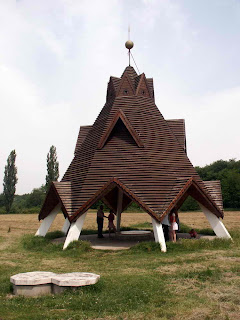## Saturday, 26 December 2009

### Where Is the Middle of Hungary?A recent excursion to the centre of historical Hungary in Szarvas aroused my curiosity and I decided to find out when and by what calculation this place was declared to be the centre of the Hungarian Kingdom. It was only after lengthy searching and with a combined utilization of Google and the New Révai Encyclopaedia (Révai Új Lexikona) that I found the following quotation from the 1879/8 issue of Vasárnapi Újság (Sunday News), a popular weekly journal of the time:

"Hungary's centre. In his work about The Natural Conditions of the Hungarian Empire ("Magyarország természeti viszonyainak leirása", Pest, 1863-65) János Hunfalvy writes: 'Hungary’s middle meridian is 38 degrees 13 minutes Eastern longitude, while its middle parallel of latitude is 46 degrees 53 minutes Northern latitude; these circles of longitude and latitude cross each other not near to Kecskemét but to Szarvas. It means that Szarvas should be approximately in the middle of the country.'In the Szarvas News ("Szarvasi Ujság"), the article continues, József Mihályfi is now giving an answer to the not uninteresting question as to where these lines exactly cross each other, where the centre of the country actually is. If these lines are drawn on the most accurate and detailed plan, which currently exists, on the sheet of Vienna’s Generalstab showing the area around Szarvas, it will become visible that that point corresponds to Kreszan’s mill, one of the two windmills built just north of the town on the area called 'green field’. So Kreszan’s windmill marks Hungary's centre."

The method was obviously rather primitive: The alleged centroid was simply determined by taking the mean value of the coordinates of latitude of the northernmost and southernmost and the coordinates of longitude of the easternmost and westernmost points of the country. The text also answers to the question of why the place is still marked by a windmill.Now, let us have a look at why Pusztavacs is regarded as the geometric centre of today's smaller country: "The landmark of Pusztavacs was built in the middle of Hungary, more specifically, at the geometric centre of the country. That is why it is worth visiting it and not for the view for example. The point marking the geometric center of our country of 93 000 km2 is situated in the north-eastern outskirts of the village. The landmark is located exactly in the intersection of the diagonals of a rectangle enclosed by” the coordinate lines of the outermost points of the country.

This is exactly the same dilettante method, only in different words. Its biggest mistake is that it has nothing to do with geometric centre. It could be called "geographic centre" at the best.

An essential feature of geometric centre or centre of gravity is namely that it does not depend on the coordinate system applied, but does depend on the shape of the figure. In fact it is defined as the intersection of the lines that divide the figure into two equal areas. The centre of gravity of a triangle is determined by the intersection of its medians, whereas that of more complex polygons can be calculated by dividing them into triangles, or by applying the following formula of analytic geometry:
s.x = sum ((v [i]. y - v [i +1]. y) * ((v [i]. x * v [i]. x) + v [i]. x * v [i + 1]. x + (v [i +1]. x * v [i +1]. x)) / (6 * T));
s.y = sum ((v [i +1]. x - v [i]. x) * ((v [i]. y * v [i]. y) + v [i]. y * v [i + 1]. y + (v [i +1]. y * v [i +1]. y)) / (6 * T));
where the coordinates of vertices and the area of the polygon are given.Although in the case of curved lines already integral calculus is needed, the centre of gravity of Hungary (or any other country) can easily be found out even at home. All you have to do is to cut the figure of the country out of cardboard, to hang it up at one point, and, as soon as it has reached the equilibrium position, to draw a vertical line downwards from the point of suspension, then to repeat the same process with another suspension. Since both lines divide the figure into equal parts, the intersection of them will show the centre of gravity. Assuming that the cardboard figure reflects the shape of the country in a fairly accurate way (and of course that the country is considered a plane figure instead of a piece of spherical surface) the true geometric center can be determined by projecting your result onto a map.

My revolutionary discovery is a bit detracted only by the fact that in the case of Germany - where both data are available – the geographical points resulting from the primitive method of bisecting the coordinates on the one hand, and from the more sophisticated calculation of centroid on the other hand, are located only 4.5 kms apart.